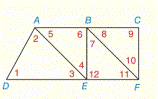Chapter 2.CR, Problem 7CR### Elementary Geometry for College St...

6th Edition
Daniel C. Alexander + 1 other
ISBN: 9781285195698

#### Solutions

Chapter
Section### Elementary Geometry for College St...

6th Edition
Daniel C. Alexander + 1 other
ISBN: 9781285195698
Textbook Problem
1 views

# For Review Exercises 7 to 11 use the given information to name the segments that must be parallel. If there are no such segments, write “none.” Assume A-B-C and D-E-F.(use the drawing from Exercise 6.) ∠ 3 ≅ ∠ 11To determine

To find:

The parallel line segments.

Explanation

Given:

3 is congruent to 11.

Figure (1)

Property:

If two lines are cut by transversal so that two corresponding angles are congruent, then the lines are parallel.

Approach:

3 is congruent to 11.

Lines AE¯ and BF

### Still sussing out bartleby?

Check out a sample textbook solution.

See a sample solution

#### The Solution to Your Study Problems

Bartleby provides explanations to thousands of textbook problems written by our experts, many with advanced degrees!

Get Started

#### Find a formula for f(n)(x) if f(x) = ln(x 1).

Single Variable Calculus: Early Transcendentals, Volume I

#### Evaluate the expression sin Exercises 116. (23)2

Finite Mathematics and Applied Calculus (MindTap Course List)

#### What is the general purpose of a manipulation check?

Research Methods for the Behavioral Sciences (MindTap Course List)